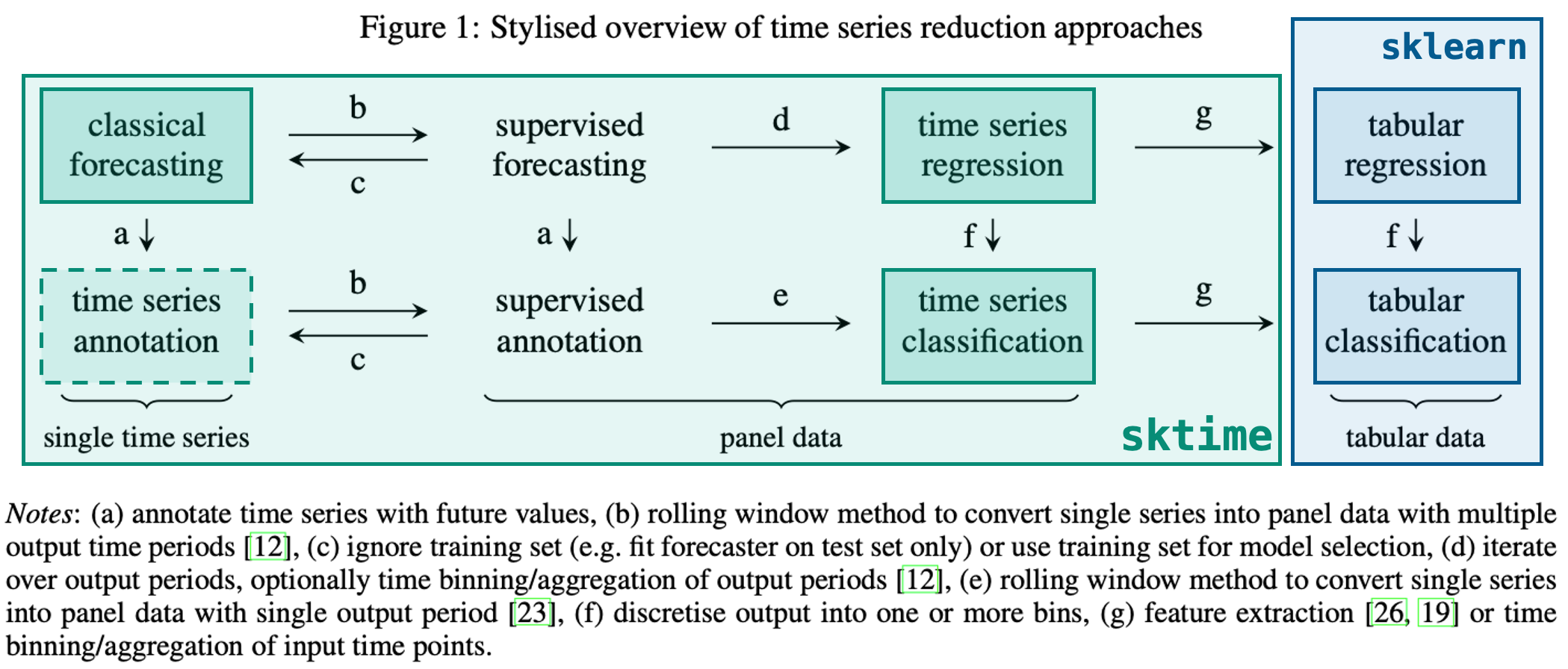# Time Series Classification

Created: Time Series Classification with SKTime and SKLearn

View this Notebook on Kaggle

# Time Series Classification with SKTime

SKTime Docs## Resources

!pip install sktime


# Methodology

Using sktime for classification is similar to using it for forecasting wherein there are either predefined models or we can transform exising sklearn models to make them usable with time series data

## Importing Data

We can import the arrow head dataset and graph some of the entries

import pandas as pd

from sktime.utils.plotting import plot_series
from sklearn.model_selection import train_test_split


X, y = load_arrow_head()

X.head()

dim_0
0 0 -1.963009 1 -1.957825 2 -1.95614...
1 0 -1.774571 1 -1.774036 2 -1.77658...
2 0 -1.866021 1 -1.841991 2 -1.83502...
3 0 -2.073758 1 -2.073301 2 -2.04460...
4 0 -1.746255 1 -1.741263 2 -1.72274...
y[:5]

array(['0', '1', '2', '0', '1'], dtype='<U1')

X_0 = list(X['dim_0'])
plot_series(pd.Series(X_0))

(<Figure size 1152x288 with 1 Axes>, <AxesSubplot:>)

<Figure size 1152x288 with 1 Axes>X_1 = list(X['dim_0'])
plot_series(pd.Series(X_1))

(<Figure size 1152x288 with 1 Axes>, <AxesSubplot:>)

<Figure size 1152x288 with 1 Axes>## Train/Test Split

Train/Test splitting can be cone using sklearn as normal since each row is a different series/observation

from sklearn.model_selection import train_test_split

X_train, X_test, y_train, y_test = train_test_split(X, y)


## Using a Classifier

sktime has built in classifiers that can be used as normal sklearn classifiers:

from sktime.classification.interval_based import TimeSeriesForestClassifier

classifier = TimeSeriesForestClassifier()
classifier.fit(X_train, y_train)

TimeSeriesForestClassifier()


And predictions can be made using the predict method:

y_pred = classifier.predict(X_test)


## Model Evaluation

We can also check the accuracy using normal sklearn metrics, for example accuracy_score

from sklearn.metrics import accuracy_score
accuracy_score(y_test, y_pred)

0.9056603773584906

from sklearn.metrics import confusion_matrix
from sklearn.metrics import confusion_matrix, ConfusionMatrixDisplay

matrix = confusion_matrix(y_test, y_pred)
disp = ConfusionMatrixDisplay(matrix)
disp.plot()

<sklearn.metrics._plot.confusion_matrix.ConfusionMatrixDisplay at 0x7f826205ddd0>

<Figure size 432x288 with 2 Axes>## Use with SKLearn Classifiers

sktime also allows the conversion of data such that it can be used with sklearn tabular classifiers. This is done by transforming the classifier using the Tabularizer in a sklearn pipeline

from sklearn.ensemble import GradientBoostingClassifier
from sklearn.pipeline import make_pipeline

from sktime.transformations.panel.reduce import Tabularizer

classifier = make_pipeline(Tabularizer(), GradientBoostingClassifier())
classifier.fit(X_train, y_train)

Pipeline(steps=[('tabularizer', Tabularizer()),

y_pred = classifier.predict(X_test)

accuracy_score(y_test, y_pred)

0.9056603773584906

matrix = confusion_matrix(y_test, y_pred)
disp = ConfusionMatrixDisplay(matrix)
disp.plot()

<sklearn.metrics._plot.confusion_matrix.ConfusionMatrixDisplay at 0x7f8261ebb810>

<Figure size 432x288 with 2 Axes>